International
Tables for
Crystallography
Volume F
Crystallography of biological macromolecules
Edited by M. G. Rossmann and E. Arnold

International Tables for Crystallography (2006). Vol. F, ch. 2.1, p. 53   | 1 | 2 |

Section 2.1.4.2. Scattering by a system of two electrons

J. Drentha*

aLaboratory of Biophysical Chemistry, University of Groningen, Nijenborgh 4, 9747 AG Groningen, The Netherlands
Correspondence e-mail: j.drenth@chem.rug.nl

2.1.4.2. Scattering by a system of two electrons

| top | pdf |

This can be derived along classical lines by calculating the phase difference between the X-ray beams scattered by each of the two electrons. A derivation based on quantum mechanics leads exactly to the same result by calculating the transition probability for the scattering of a primary quantum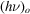, given a secondary quantum(Heitler, 1966, p. 193). For simplification we shall give only the classical derivation here. In Fig. 2.1.4.2,a system of two electrons is drawn with the origin at electron 1 and electron 2 at position r. They scatter the incident beam in a direction given by the vector s. The direction of the incident beam is along the vector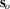. The length of the vectors can be chosen arbitrarily, but for convenience they are given a length. The two electrons scatter completely independently of each other.Figure 2.1.4.2 | top | pdf |The black dots are electrons. The origin of the system is at electron 1; electron 2 is at position r. The electrons are irradiated by an X-ray beam from the direction indicated by vector. The radiation scattered by the electrons is observed in the direction of vector s. Because of the path difference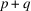, scattered beam 2 will lag behind scattered beam 1 in phase. Reproduced with permission from Drenth (1999). Copyright (1999) Springer-Verlag.

Therefore, the amplitudes of the scattered beams 1 and 2 are equal, but they have a phase difference resulting from the path difference between the beam passing through electron 2 and the beam passing through electron 1. The path difference is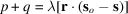. Beam 2 lags behind in phase compared with beam 1, and with respect to wave 1 its phase angle is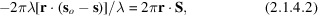where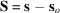.

From Fig. 2.1.4.3,it is clear that the direction of S is perpendicular to an imaginary plane reflecting the incident beam at an angle θ and that the length of S is given by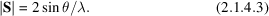The total scattering from the two-electron system is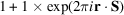if the resultant amplitude of the waves from electrons 1 and 2 is set to 1. In an Argand diagram, the waves are represented by vectors in a two-dimensional plane, as in Fig. 2.1.4.4(a).1 Thus far, the origin of the system was chosen at electron 1. Moving the origin to another position simply means an equal change of phase angle for all waves. Neither the amplitudes nor the intensities of the reflected beams change (Fig. 2.1.4.4b).Figure 2.1.4.3 | top | pdf |The direction of the incident wave is indicated byand that of the scattered wave by s. Both vectors are of length. A plane that makes equal angles with s andcan be regarded as a mirror reflecting the incident beam. Reproduced with permission from Drenth (1999). Copyright (1999) Springer-Verlag.Figure 2.1.4.4 | top | pdf |An Argand diagram for the scattering by two electrons. In (a), the origin is at electron 1; electron 2 is at position r with respect to electron 1. In (b), electron 1 is at position R with respect to the new origin, and electron 2 is at position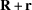.

References

Heitler, W. G. (1966). The quantum theory of radiation, 3rd ed. Oxford University Press.